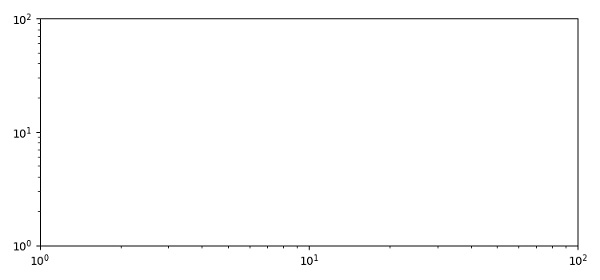# Matplotlib log scale tick label number formatting

To set the log scale tick label number on the axes, we can take the following steps −

• Set x and y axis limits (1 to 100), using ylim and xlim, on both the axes.

• Using loglog() method, make a plot with log scaling on both the x and y axis.

• To display the figure, use the plot() method.

## Example

from matplotlib import pyplot as plt
plt.rcParams["figure.figsize"] = [7.50, 3.50]
plt.rcParams["figure.autolayout"] = True
plt.ylim(1, 100)
plt.xlim(1, 100)
plt.loglog()
plt.show()

## Output